“中国国际名城经典车拉力赛2017” 北京站完美落幕

2017年9月17日，随着来自世界各地、代表着汽车工业各时期的近40辆经典车列队徐徐驶入张家口市民广场，第四届“中国国际名城经典车拉力赛”暂告一段落。在这为期三天精彩纷呈的比赛中，一辆辆宛如艺术品般的经典车从司马台长城脚下独具北方特色的度假胜地古北水镇出发，漫游在这金秋时节的京畿大地上，不仅让参赛选手们留下了美好的回忆，也向广大民众生动地传播了经典车文化。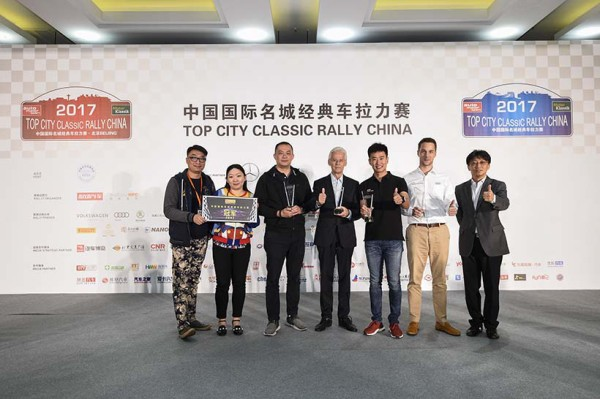今年的“中国国际名城经典车拉力赛”车型之多、收藏价值之高可谓历届赛事之最。尤其是作为本次赛事的首席战略合作伙伴，梅赛德斯-奔驰在新一代S级即将登陆中国市场之际，齐聚S级家族从1951年伊始先后诞生的9代成员。这个奔驰的旗舰车型系列自始至终都将“世界上最好的汽车”作为自己的定位。而奔驰还将珍藏于斯图加特奔驰博物馆的第二代奔驰S级（代号为W180）和第三代奔驰S级（代号为W111）带到中国参赛，让更多人能够一睹这两款稀有而经典的汽车。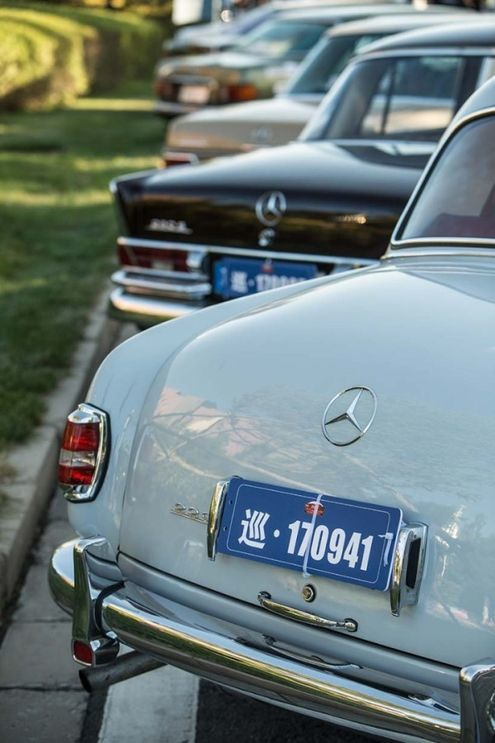在北京站的比赛中，S级证明了它们应有的实力——由北京梅赛德斯-奔驰销售服务有限公司售后服务部保修、善意维修和服务合约总监Christian Schroeter先生，和德国MPS集团出版人Bernd Ostmann先生驾驶的第三代S级：奔驰230S获得了总分第一的好成绩。这辆1965年制造的230S除了外观完好，机械也都保持在近乎于完美的状态。而戴姆勒股份有限公司董事会成员唐仕凯（Hubertus Troska）先生和北京梅赛德斯-奔驰销售服务有限公司公共关系及媒体传播总监官少卿先生驾驶的第二代S级220a也斩获了第七名。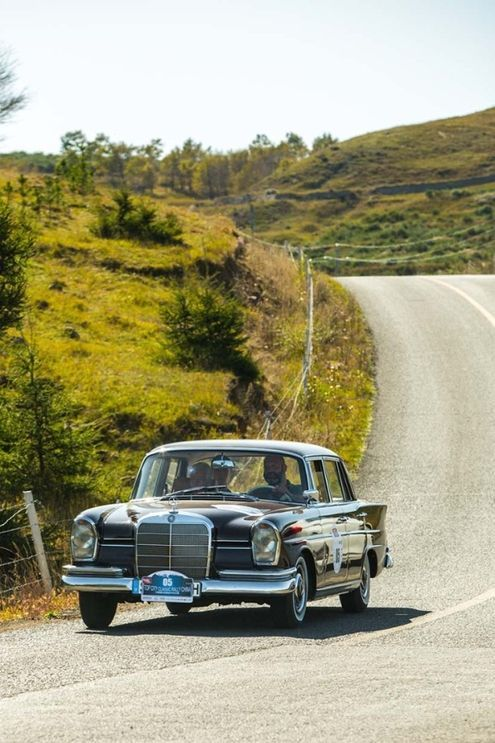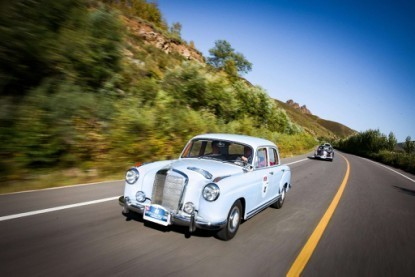另外，梅赛德斯-奔驰还获得了“中国国际名城经典车拉力赛2017”北京站的经典大奖和经典汽车运动大奖，S级轿车则是荣获了传奇大奖。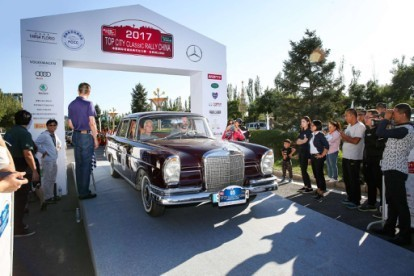同样来自德国的参赛者还有大众汽车集团，奥迪、斯柯达、保时捷以及大众汽车品牌的参与让它的比赛车型更丰富多彩。1984年世界拉力锦标赛中一鸣惊人的Audi Rally quattro A2赛车今天在奥迪著名车手程丛夫和其它奥迪车手的驾驭下，成功获得总成绩亚军，并成为本次优雅跑车大奖的得主。奥迪A6的前身：奥迪100（C3）也进入了前八名，并且获驾驶乐趣大奖。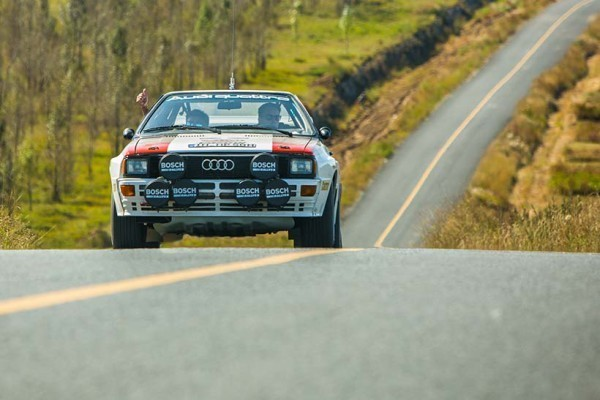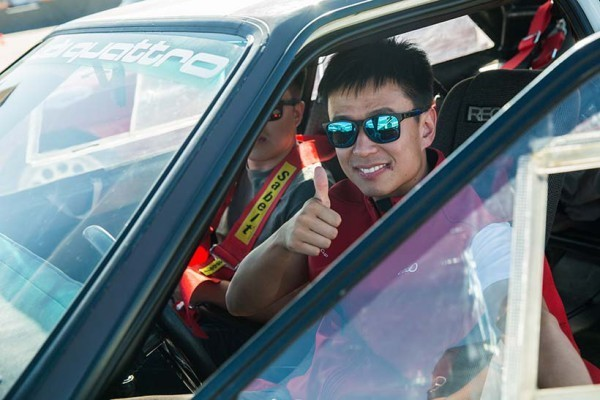拥有122年历史的斯柯达则带来了上世纪50年代曾经风靡欧洲的Felicia，这款车身轻盈、造型美观的敞篷跑车吸引了最多的目光。而本届比赛中最古老的斯柯达422也在人们的精心维护下，顺利地完成了比赛，还获得了本站比赛的风尚大奖。50年代初问世的首脑座驾VOS则得到了历史重现大奖。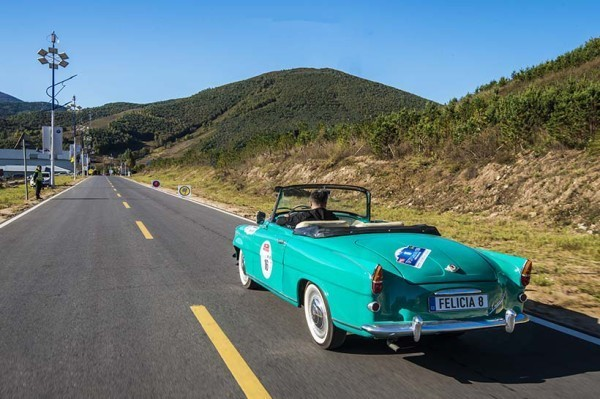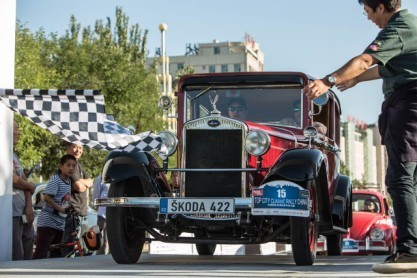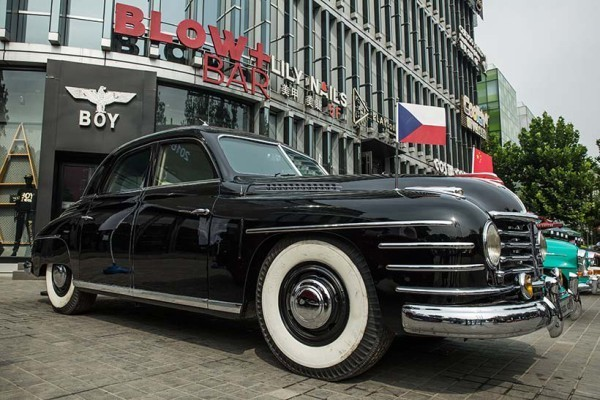大众汽车T3、甲壳虫、保时捷914和911等车型风采各异，充分体现了大众汽车集团的悠久历史和丰富车型。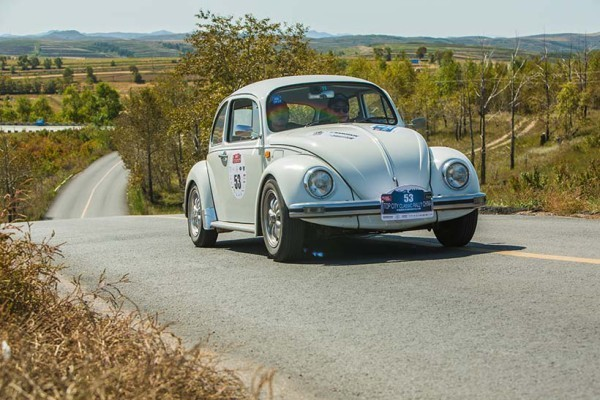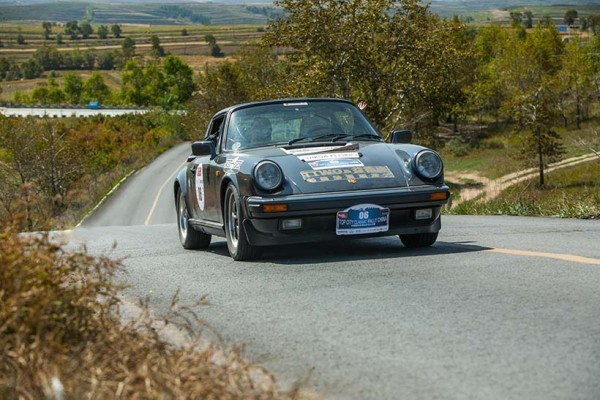除了汽车厂家提供的车辆，本届比赛还吸引了众多的经典车藏家和收藏机构。其中最具代表性的当属中国著名经典车收藏家、经典车文化委员会副主任、大连经典汽车文化中心、大连时空宝库经典汽车博物馆创始人韩业广先生带来的福特A型车。这款车产于1930年，它的造型富有韵味、性能出色，时至今日仍能顺利完成比赛，并取得了优秀的成绩。当然，参赛车辆的表现离不开本届赛事的独家轮胎赞助商倍耐力，由其提供的高性能轮胎为这些经典车辆性能的发挥奠定了基础。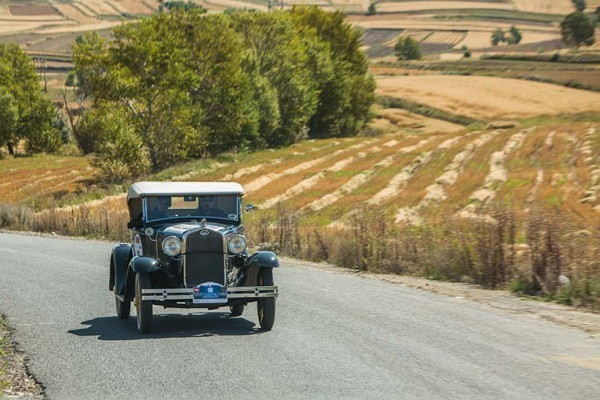在张家口独家赞助酒店，密苑云顶酒店举办的“中国国际名城经典车拉力赛2017”北京站颁奖晚宴上，主办方将经典车运动贡献奖颁发给了张家口市经济开发区，张家口经开区副主任丁凡、张家口经开区文体局副局长聂丽霞作为代表接过了奖杯。张家口作为2022年冬奥会的共同主办城市，文体事业正在迅猛发展，“中国国际名城经典车拉力赛”与张家口结缘，形成了真正的双赢局面，创造了良好的社会文化效益。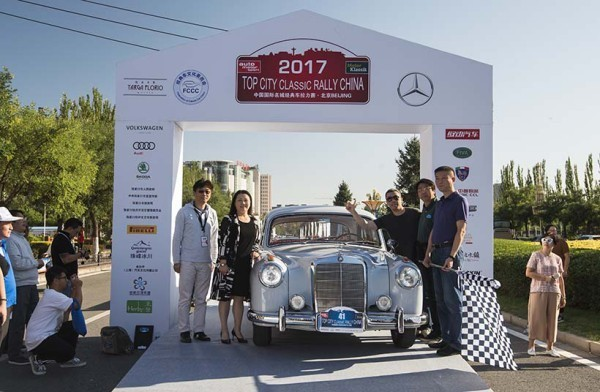随着本届比赛北京站的正式落幕，“中国国际名城经典车拉力赛2017”即将移师上海，在中都物流的鼎力支持下，将于9月21日在东方明珠电视塔脚下展开静展，并于接下来的两天开始上海站的角逐，让我们共同期盼本届比赛的新高潮！

##### 相关资讯

|分享

•已阅！握爪
•我手滑为你点赞
•128个赞！
•不明觉厉
•阅后既醉
•有钱！任性
•照片太美，我不敢看
•干货！杠杠的
•高大上
•膜拜中！
•请接受我的膝盖
•猫哥/猫妹么么哒
•神吐槽
•我只笑笑不说话
•我想静静
•窒息
##### 资讯相关车系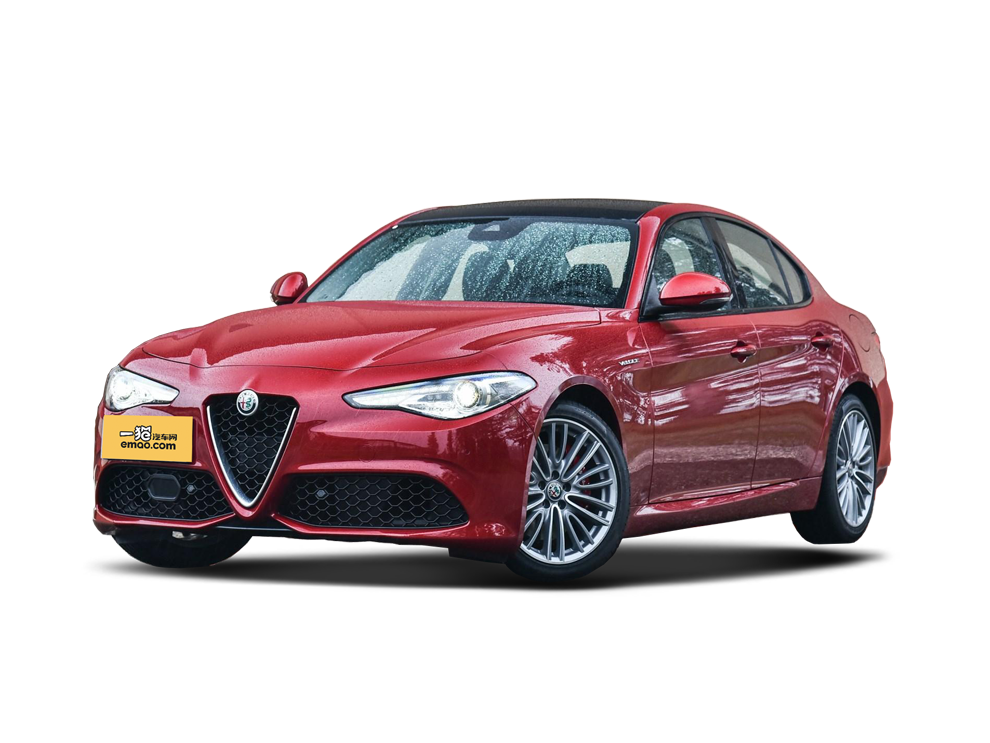##### 热度排行• 阿斯顿·马丁
• 奥迪
• 阿尔法罗密欧
• ALPINA
• 爱驰
• AC Schnitzer

• 巴博斯
• 宝骏
• 宝马
• 保时捷
• 北汽制造
• 奔驰
• 奔腾
• 本田
• 比亚迪
• 标致
• 别克
• 宾利
• 布加迪
• 北汽威旺
• 北京
• 北汽绅宝
• 北汽幻速
• 北汽新能源
• 宝沃
• 比速汽车
• 北汽道达
• 北京汽车
• 比德文汽车
• 铂驰
• 北汽昌河

• 昌河
• 长安
• 长城
• 长安商用
• 成功汽车
• 长江EV
• 长安轻车型
• 长安欧尚
• 长安凯程
• 长安跨越
• 车驰汽车

• 大众
• 道奇
• 东风
• 东风风神
• 东风小康
• 东南
• DS
• 东风风行
• 东风风度
• 东风风光
• 电咖
• 大乘汽车
• 大运
• 东风富康

• 法拉利
• 菲亚特
• 丰田
• 福迪
• 福特
• 福田汽车
• 福汽启腾
• 风诺
• 福田

• GMC
• 广汽传祺
• 广汽吉奥
• 观致
• 国金汽车
• 广汽新能源
• 广汽集团
• 国机智骏

• 哈飞
• 海格
• 海马
• 华泰
• 黄海
• 恒天
• 红旗
• 哈弗
• 华颂
• 华凯
• 华泰新能源
• 汉腾汽车
• 华利
• 红星汽车
• HYCAN合创
• 汉龙汽车

• Jeep
• 江淮
• 江铃
• 捷豹
• 金杯
• 九龙
• 吉利汽车
• 金旅
• 金龙
• 江铃集团轻汽
• 江铃集团新能源
• 君马汽车
• 捷途
• 钧天
• 捷达
• 几何汽车

• 开瑞
• 凯迪拉克
• 科尼赛克
• 克莱斯勒
• KTM
• 卡威
• 凯翼
• 康迪
• 康迪电动汽车集团
• 开沃汽车
• 卡升

• 猎豹汽车
• 兰博基尼
• 劳斯莱斯
• 雷克萨斯
• 雷诺
• 理念
• 力帆
• 莲花汽车
• 林肯
• 铃木
• 陆风
• 路虎
• 路特斯
• 领志
• 领克
• 零跑汽车
• Lorinser
• LOCAL MOTORS
• LITE
• 领途汽车
• 理想汽车
• 罗夫哈特
• 力帆汽车

• MG
• MINI
• 马自达
• 玛莎拉蒂
• 迈凯伦
• 摩根
• 名爵
• 迈莎锐
• 迈迈

• 纳智捷
• 哪吒汽车

• 讴歌
• 欧宝
• 欧朗
• 欧拉
• 欧尚汽车

• 帕加尼
• Polestar极星

• 奇瑞
• 启辰
• 起亚
• 前途
• 庆铃汽车
• 骐铃汽车
• 全球鹰
• 乔治·巴顿

• 日产
• 荣威
• 瑞麒汽车
• 如虎
• 瑞驰
• 瑞麒
• 瑞驰新能源
• 容大智造

• 上汽大通
• smart
• 三菱
• 双环
• 双龙
• 斯巴鲁
• 斯柯达
• 萨博
• 思铭
• 赛麟
• SWM斯威汽车
• 上汽MAXUS
• SRM鑫源
• 思皓
• SHELBY
• 上喆

• TESLA
• 泰卡特
• 腾势
• 特斯拉
• 天际汽车

• 威麟
• 威兹曼
• 沃尔沃
• 五菱汽车
• 五十铃
• 潍柴英致
• WEY
• 蔚来
• 威马汽车
• 潍柴汽车

• 现代
• 雪佛兰
• 雪铁龙
• 西雅特
• 新特汽车
• 小鹏汽车
• 新宝骏

• 野马汽车
• 一汽
• 依维柯
• 英菲尼迪
• 永源
• 驭胜
• 云度
• 宇通客车
• 云雀汽车
• 远程汽车
• 一汽凌河

• 中华
• 中兴
• 众泰
• 知豆
• 之诺
• 正道汽车
• A
• B
• C
• D
• E
• F
• G
• H
• I
• J
• K
• L
• M
• N
• O
• P
• Q
• R
• S
• T
• U
• V
• W
• X
• Y
• Z

• 阿斯顿·马丁
• 奥迪
• 阿尔法罗密欧
• ALPINA
• 爱驰
• AC Schnitzer

• 巴博斯
• 宝骏
• 宝马
• 保时捷
• 北汽制造
• 奔驰
• 奔腾
• 本田
• 比亚迪
• 标致
• 别克
• 宾利
• 布加迪
• 北汽威旺
• 北京
• 北汽绅宝
• 北汽幻速
• 北汽新能源
• 宝沃
• 比速汽车
• 北汽道达
• 北京汽车
• 比德文汽车
• 铂驰
• 北汽昌河

• 昌河
• 长安
• 长城
• 长安商用
• 成功汽车
• 长江EV
• 长安轻车型
• 长安欧尚
• 长安凯程
• 长安跨越
• 车驰汽车

• 大众
• 道奇
• 东风
• 东风风神
• 东风小康
• 东南
• DS
• 东风风行
• 东风风度
• 东风风光
• 电咖
• 大乘汽车
• 大运
• 东风富康

• 法拉利
• 菲亚特
• 丰田
• 福迪
• 福特
• 福田汽车
• 福汽启腾
• 风诺
• 福田

• GMC
• 广汽传祺
• 广汽吉奥
• 观致
• 国金汽车
• 广汽新能源
• 广汽集团
• 国机智骏

• 哈飞
• 海格
• 海马
• 华泰
• 黄海
• 恒天
• 红旗
• 哈弗
• 华颂
• 华凯
• 华泰新能源
• 汉腾汽车
• 华利
• 红星汽车
• HYCAN合创
• 汉龙汽车

• Jeep
• 江淮
• 江铃
• 捷豹
• 金杯
• 九龙
• 吉利汽车
• 金旅
• 金龙
• 江铃集团轻汽
• 江铃集团新能源
• 君马汽车
• 捷途
• 钧天
• 捷达
• 几何汽车

• 开瑞
• 凯迪拉克
• 科尼赛克
• 克莱斯勒
• KTM
• 卡威
• 凯翼
• 康迪
• 康迪电动汽车集团
• 开沃汽车
• 卡升

• 猎豹汽车
• 兰博基尼
• 劳斯莱斯
• 雷克萨斯
• 雷诺
• 理念
• 力帆
• 莲花汽车
• 林肯
• 铃木
• 陆风
• 路虎
• 路特斯
• 领志
• 领克
• 零跑汽车
• Lorinser
• LOCAL MOTORS
• LITE
• 领途汽车
• 理想汽车
• 罗夫哈特
• 力帆汽车

• MG
• MINI
• 马自达
• 玛莎拉蒂
• 迈凯伦
• 摩根
• 名爵
• 迈莎锐
• 迈迈

• 纳智捷
• 哪吒汽车

• 讴歌
• 欧宝
• 欧朗
• 欧拉
• 欧尚汽车

• 帕加尼
• Polestar极星

• 奇瑞
• 启辰
• 起亚
• 前途
• 庆铃汽车
• 骐铃汽车
• 全球鹰
• 乔治·巴顿

• 日产
• 荣威
• 瑞麒汽车
• 如虎
• 瑞驰
• 瑞麒
• 瑞驰新能源
• 容大智造

• 上汽大通
• smart
• 三菱
• 双环
• 双龙
• 斯巴鲁
• 斯柯达
• 萨博
• 思铭
• 赛麟
• SWM斯威汽车
• 上汽MAXUS
• SRM鑫源
• 思皓
• SHELBY
• 上喆

• TESLA
• 泰卡特
• 腾势
• 特斯拉
• 天际汽车

• 威麟
• 威兹曼
• 沃尔沃
• 五菱汽车
• 五十铃
• 潍柴英致
• WEY
• 蔚来
• 威马汽车
• 潍柴汽车

• 现代
• 雪佛兰
• 雪铁龙
• 西雅特
• 新特汽车
• 小鹏汽车
• 新宝骏

• 野马汽车
• 一汽
• 依维柯
• 英菲尼迪
• 永源
• 驭胜
• 云度
• 宇通客车
• 云雀汽车
• 远程汽车
• 一汽凌河

• 中华
• 中兴
• 众泰
• 知豆
• 之诺
• 正道汽车
• A
• B
• C
• D
• E
• F
• G
• H
• I
• J
• K
• L
• M
• N
• O
• P
• Q
• R
• S
• T
• U
• V
• W
• X
• Y
• Z

• 阿斯顿·马丁
• 奥迪
• 阿尔法罗密欧
• ALPINA
• 爱驰
• AC Schnitzer

• 巴博斯
• 宝骏
• 宝马
• 保时捷
• 北汽制造
• 奔驰
• 奔腾
• 本田
• 比亚迪
• 标致
• 别克
• 宾利
• 布加迪
• 北汽威旺
• 北京
• 北汽绅宝
• 北汽幻速
• 北汽新能源
• 宝沃
• 比速汽车
• 北汽道达
• 北京汽车
• 比德文汽车
• 铂驰
• 北汽昌河

• 昌河
• 长安
• 长城
• 长安商用
• 成功汽车
• 长江EV
• 长安轻车型
• 长安欧尚
• 长安凯程
• 长安跨越
• 车驰汽车

• 大众
• 道奇
• 东风
• 东风风神
• 东风小康
• 东南
• DS
• 东风风行
• 东风风度
• 东风风光
• 电咖
• 大乘汽车
• 大运
• 东风富康

• 法拉利
• 菲亚特
• 丰田
• 福迪
• 福特
• 福田汽车
• 福汽启腾
• 风诺
• 福田

• GMC
• 广汽传祺
• 广汽吉奥
• 观致
• 国金汽车
• 广汽新能源
• 广汽集团
• 国机智骏

• 哈飞
• 海格
• 海马
• 华泰
• 黄海
• 恒天
• 红旗
• 哈弗
• 华颂
• 华凯
• 华泰新能源
• 汉腾汽车
• 华利
• 红星汽车
• HYCAN合创
• 汉龙汽车

• Jeep
• 江淮
• 江铃
• 捷豹
• 金杯
• 九龙
• 吉利汽车
• 金旅
• 金龙
• 江铃集团轻汽
• 江铃集团新能源
• 君马汽车
• 捷途
• 钧天
• 捷达
• 几何汽车

• 开瑞
• 凯迪拉克
• 科尼赛克
• 克莱斯勒
• KTM
• 卡威
• 凯翼
• 康迪
• 康迪电动汽车集团
• 开沃汽车
• 卡升

• 猎豹汽车
• 兰博基尼
• 劳斯莱斯
• 雷克萨斯
• 雷诺
• 理念
• 力帆
• 莲花汽车
• 林肯
• 铃木
• 陆风
• 路虎
• 路特斯
• 领志
• 领克
• 零跑汽车
• Lorinser
• LOCAL MOTORS
• LITE
• 领途汽车
• 理想汽车
• 罗夫哈特
• 力帆汽车

• MG
• MINI
• 马自达
• 玛莎拉蒂
• 迈凯伦
• 摩根
• 名爵
• 迈莎锐
• 迈迈

• 纳智捷
• 哪吒汽车

• 讴歌
• 欧宝
• 欧朗
• 欧拉
• 欧尚汽车

• 帕加尼
• Polestar极星

• 奇瑞
• 启辰
• 起亚
• 前途
• 庆铃汽车
• 骐铃汽车
• 全球鹰
• 乔治·巴顿

• 日产
• 荣威
• 瑞麒汽车
• 如虎
• 瑞驰
• 瑞麒
• 瑞驰新能源
• 容大智造

• 上汽大通
• smart
• 三菱
• 双环
• 双龙
• 斯巴鲁
• 斯柯达
• 萨博
• 思铭
• 赛麟
• SWM斯威汽车
• 上汽MAXUS
• SRM鑫源
• 思皓
• SHELBY
• 上喆

• TESLA
• 泰卡特
• 腾势
• 特斯拉
• 天际汽车

• 威麟
• 威兹曼
• 沃尔沃
• 五菱汽车
• 五十铃
• 潍柴英致
• WEY
• 蔚来
• 威马汽车
• 潍柴汽车

• 现代
• 雪佛兰
• 雪铁龙
• 西雅特
• 新特汽车
• 小鹏汽车
• 新宝骏

• 野马汽车
• 一汽
• 依维柯
• 英菲尼迪
• 永源
• 驭胜
• 云度
• 宇通客车
• 云雀汽车
• 远程汽车
• 一汽凌河

• 中华
• 中兴
• 众泰
• 知豆
• 之诺
• 正道汽车
• A
• B
• C
• D
• E
• F
• G
• H
• I
• J
• K
• L
• M
• N
• O
• P
• Q
• R
• S
• T
• U
• V
• W
• X
• Y
• Z